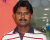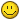# 0 Decimal Points In Y-Axis In Mscolumn2D.swf

## Recommended Posts

I am working with some older version of Fusion Charts (not v3.2). I am using MSColumn2D.swf to render the graph.

My requirement is to display one decimal values in Y-Axis. This is working fine for decimals other than zero. For example if the number is 10 or 10.0 I would like to display 10.0 only in Y-Axis. But with the older version it is displaying as 10 not as 10.0. Can you please advise how can I fix the issue.

I even tried the below chart attributes but it is not working.

<chart yAxisMinValue='115' yAxisMaxValue='140' adjustDiv='0' numDivLines='3' forceYAxisValueDecimals='1' yAxisValueDecimals='2' decimals='0' >

Thanks

##### Share on other sitesHi,

Please find below the modified chart attributes:

<chart .. .. yAxisMinValue='115' yAxisMaxValue='140' adjustDiv='0' numDivLines='3' forceYAxisValueDecimals='1' yAxisValueDecimals='1' decimals='1' formatNumberScale='0' .. .. >

Hope this helps.##### Share on other sites

But the code you suggested still does not show zero decimal. I am using the below xml for old version of fusion chart.

<chart yAxisMinValue='115' yAxisMaxValue='140' adjustDiv='0' numDivLines='3' forceYAxisValueDecimals='1' yAxisValueDecimals='1' decimals='1' formatNumberScale='0'>

<categories>

<category label="1-Year"/>

<category label="3-Year"/>

<category label="5-Year"/>

</categories>

<dataset seriesName="AA" color="669900" >

<set value="45.5" displayvalue='45.5%' color="#c0504d"/>

<set value="13.3" displayvalue='13.3%' color="#c0504d"/>

<set value="7.8" displayvalue='7.8%' color="#c0504d"/>

</dataset>

<dataset seriesName="Peer Median" color="3399FF" >

<set value="47.2" displayvalue='47.2%'/>

<set value="13.8" displayvalue='13.8%'/>

<set value="8.0" displayvalue='8.0%'/>

</dataset>

<dataset seriesName="Industry Median" color="454545" >

<set value="40.0" displayvalue='40.0%'/>

<set value="-8.6" displayvalue='-8.6%'/>

<set value="0.0" displayvalue='0.0%'/>

</dataset>

</chart>

Edited by venkatc

##### Share on other sitesHi,

To update you, this Issue has been fixed in FusionCharts v3.2.

I hope this helps.